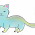### What's Wrong? Q8b

1.he should do the ^2 1st and then change the denominator

2.The student skipped step. I think...

3.Brackets must be calculated first, thus the power must be done first

4.He should expand the value in the brackets before multiplying it by the denominators to make them equal.

5.the person must square first.

6.Agree with matthew

7.erm...he was careless when doing the ^2

8.Careless...

9.The person should have done the ^2 first.

10.He skipped a step as there should be a (3+p)(3+p)/6 + (p-5)(p-5) and he also did the calculations wrongly, thus, the question went wrong from the start.

11.For the first line, he like did 3x2 instead of 3^2. Same for 2p.

12.He skipped a step. did not display the necessary steps.

13.The person did not do the ^2 outside the parenthesis but did the multiplication first.

14.He must do the ^2 before multiplying it by3

15.He should do the working in the bracket first before proceeding to make the denominator the same.

16.The brackets should be counted before the Power

17.He/She did not show his working

18.Should be squared

19.The person did the multiplication before he/she did the '^2'

20.Brackets first, before changing the denominator.

21.He should do the ^2 first

22.The student done the question in the wrong order

23.The student should also square the p.